Open in App
Not now

# Class 10 NCERT Solutions- Chapter 6 Triangles – Exercise 6.2

• Last Updated : 17 Nov, 2022

Theorem 6.1 :

If a line is drawn parallel to one side of a triangle to intersect the other two sides in distinct points, the other two sides are divided in the same ratio.

Theorem 6.2 :

If a line divides any two sides of a triangle in the same ratio, then the line is parallel to the third side.

### Question 1. In Figure, (i) and (ii), DE || BC. Find EC in (i) and AD in (ii).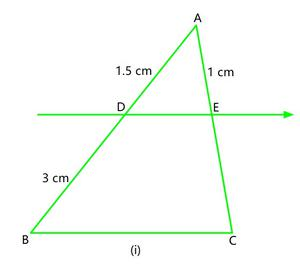Solution:

(i) Here, In △ ABC,

DE || BC

So, according to Theorem 6.1⇒EC =EC = 2 cm

Hence, EC = 2 cm.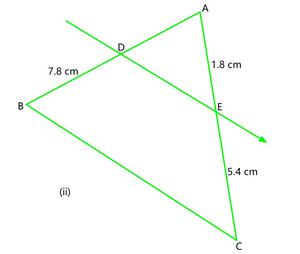(ii) Here, In △ ABC,

So, according to Theorem 6.1 , if DE || BC⇒AD =### Question 2. E and F are points on the sides PQ and PR respectively of a ∆ PQR. For each of the following cases, state whether EF || QR :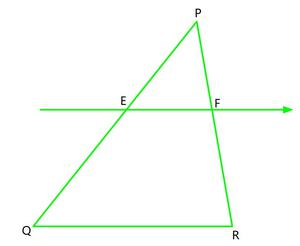### (i) PE = 3.9 cm, EQ = 3 cm, PF = 3.6 cm and FR = 2.4 cm

Solution:

According to the Theorem 6.2,

If a line divides any two sides of a triangle in the same ratio, then the line is parallel to the third side.

So, lets check the ratios

Here, In △ PQR,= 1.3 ………………………(i)= 1.5 ………………………(ii)

As,Hence, EF is not parallel to QR.

### (ii) PE = 4 cm, QE = 4.5 cm, PF = 8 cm and RF = 9 cm

Solution:

According to the Theorem 6.2,

If a line divides any two sides of a triangle in the same ratio, then the line is parallel to the third side.

So, lets check the ratios

Here, In △ PQR,………………………(i)………………………(ii)

As,Hence, EF is parallel to QR.

### (iii) PQ = 1.28 cm, PR = 2.56 cm, PE = 0.18 cm and PF = 0.36 cm

Solution:

EQ = PQ – PE = 1.28 – 0.18 = 1.1

and, FR = PR – PF = 2.56 – 0.36 = 2.2

According to the Theorem 6.2,

If a line divides any two sides of a triangle in the same ratio, then the line is parallel to the third side.

So, lets check the ratios

Here, In △ PQR,………………………(i)………………………(ii)

As,Hence, EF is parallel to QR.

###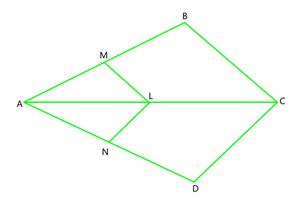Solution:

Here, In △ ABC,

According to Theorem 6.1, if LM || CB

then,…………………………….(I)

According to Theorem 6.1, if LN || CD

then,…………………………….(II)

From (I) and (II), we conclude thatHence Proved !!

###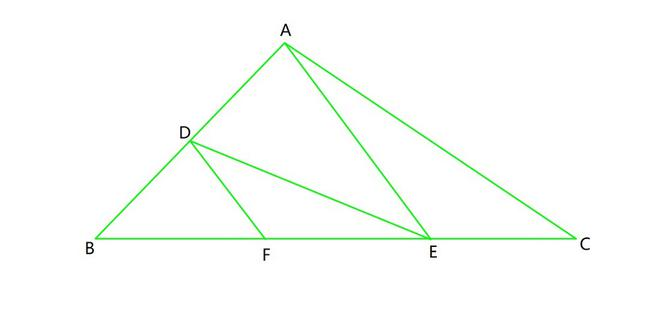Solution:

Here, In △ ABC,

According to Theorem 6.1, if DE || AC

then,…………………………….(I)

and, In △ ABE,

According to Theorem 6.1, if DF || AE

then,…………………………….(II)

From (I) and (II), we conclude thatHence Proved !!

### Question 5. In Figure, DE || OQ and DF || OR. Show that EF || QR.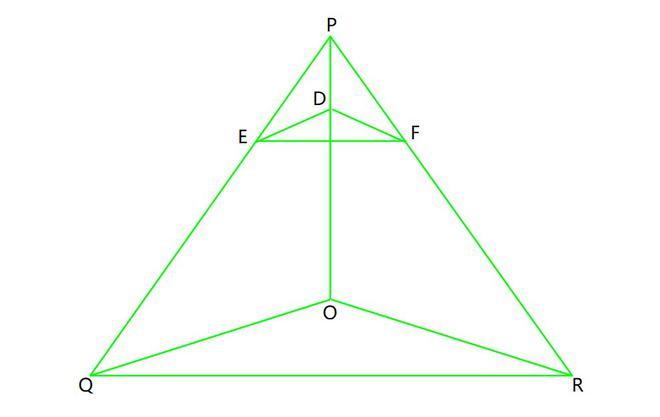Solution:

Here, In △ POQ,

According to Theorem 6.1, if DE || OQ

then,…………………………….(I)

and, In △ POR,

According to Theorem 6.1, if DF || OR

then,…………………………….(II)

From (I) and (II), we conclude that………………………………(III)

According to Theorem 6.2 and eqn. (III)

EF || QR, in △ PQR

Hence Proved !!

### Question 6. In Figure, A, B and C are points on OP, OQ and OR respectively such that AB || PQ and AC || PR. Show that BC || QR.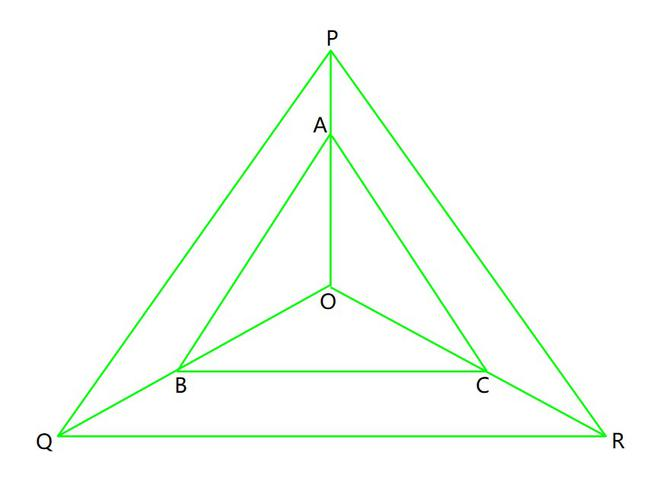Solution:

Here, In △ POQ,

According to Theorem 6.1, if AB || PQ

then,…………………………….(I)

and, In △ POR,

According to Theorem 6.1, if AC || PR

then,…………………………….(II)

From (I) and (II), we conclude that………………………………(III)

According to Theorem 6.2 and eqn. (III)

BC || QR, in △ OQR

Hence Proved !!

### Question 7. Using Theorem 6.1, prove that a line drawn through the mid-point of one side of a triangle parallel to another side bisects the third side.

Solution:

Given, in ΔABC, D is the midpoint of AB such that AD=DB.

A line parallel to BC intersects AC at E

So, DE || BC.

We have to prove that E is the mid point of AC.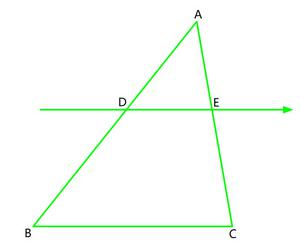= 1 …………………………. (I)

Here, In △ ABC,

According to Theorem 6.1, if DE || BC

then,…………………………….(II)

From (I) and (II), we conclude that= 1= 1

AE = EC

E is the midpoint of AC.

Hence proved !!

### Question 8. Using Theorem 6.2, prove that the line joining the mid-points of any two sides of a triangle is parallel to the third side.

Solution:

Given, in ΔABC, D and E are the mid points of AB and AC respectively

We have to prove that: DE || BC.= 1 …………………………. (I)

and, AE=EC= 1 …………………………. (II)

From (I) and (II), we conclude that= 1 ……………….(III)

According to Theorem 6.2 and eqn. (III)

DE || BC, in △ ABC

Hence Proved !!

###Solution:

From the point O, draw a line EO touching AD at E, in such a way that,

EO || DC || AB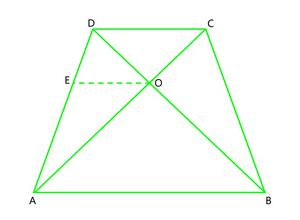According to Theorem 6.1, if AB || EO

then,…………………………….(I)

According to Theorem 6.1, if AC || PR

then,…………………………….(II)

From (I) and (II), we conclude thatAfter rearranging, we getHence Proved !!

###. Show that ABCD is a trapezium.

Solution:

From the point O, draw a line EO touching AD at E, in such a way that,

EO || DC || ABAccording to Theorem 6.1, if AB || EO

then,…………………………….(I)(Given)(After rearranging) ………………………………..(II)

From (I) and (II), we conclude that………………………………..(III)

According to Theorem 6.2 and eqn. (III)

EO || DC and also EO || AB

⇒ AB || DC.

Hence, quadrilateral ABCD is a trapezium with AB || CD.

My Personal Notes arrow_drop_up
Related Articles